Tamil Nadu Board of Secondary EducationSSLC (English Medium) Class 6th

# Tamil Nadu Board Samacheer Kalvi solutions for Class 6th Mathematics Term 2 Answers Guide chapter 5 - Information Processing [Latest edition]

#### Chapters## Chapter 5: Information Processing

Exercise 5.1Exercise 5.2
Exercise 5.1 [Page 83]

### Tamil Nadu Board Samacheer Kalvi solutions for Class 6th Mathematics Term 2 Answers Guide Chapter 5 Information Processing Exercise 5.1 [Page 83]

Exercise 5.1 | Q 1. (i) | Page 83

Convert the following numerical expression into Tree diagram.

8 + (6 × 2)

Exercise 5.1 | Q 1. (ii) | Page 83

Convert the following numerical expression into Tree diagram.

9 − (2 × 3)

Exercise 5.1 | Q 1. (iii) | Page 83

Convert the following numerical expression into Tree diagram.

(3 × 5) − (4 ÷ 2)

Exercise 5.1 | Q 1. (iv) | Page 83

Convert the following numerical expression into Tree diagram.

[(2 × 4) + 2] × (8 ÷ 2)

Exercise 5.1 | Q 1. (v) | Page 83

Convert the following numerical expression into Tree diagram.

[(6 + 4) × 7] ÷ [2 × (10 − 5)]

Exercise 5.1 | Q 1. (vi) | Page 83

Convert the following numerical expression into Tree diagram.

[(4 × 3) ÷ 2] + [8 × (5 – 3)]

Exercise 5.1 | Q 2. (i) | Page 83

Convert the following Tree diagram into numerical expression.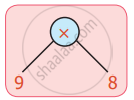Exercise 5.1 | Q 2. (ii) | Page 83

Convert the following Tree diagram into numerical expression.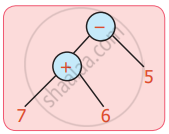Exercise 5.1 | Q 2. (iii) | Page 83

Convert the following Tree diagram into numerical expression.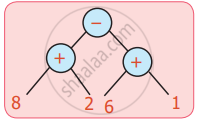Exercise 5.1 | Q 2. (iv) | Page 83

Convert the following Tree diagram into numerical expression.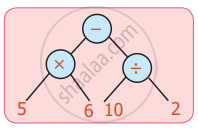Exercise 5.1 | Q 3. (i) | Page 83

Convert the following algebraic expression into tree diagram.

10v

Exercise 5.1 | Q 3. (ii) | Page 83

Convert the following algebraic expression into tree diagram.

3a – b

Exercise 5.1 | Q 3. (iii) | Page 83

Convert the following algebraic expression into tree diagram.

5x + y

Exercise 5.1 | Q 3. (iv) | Page 83

Convert the following algebraic expression into tree diagram.

20t × p

Exercise 5.1 | Q 3. (v) | Page 83

Convert the following algebraic expression into tree diagram.

2(a + b)

Exercise 5.1 | Q 3. (vi) | Page 83

Convert the following algebraic expression into tree diagram.

(x × y) – (y × z)

Exercise 5.1 | Q 3. (vii) | Page 83

Convert the following algebraic expression into tree diagram.

4x + 5y

Exercise 5.1 | Q 3. (viii) | Page 83

Convert the following algebraic expression into tree diagram.

(lm – n) ÷ (pq + r)

Exercise 5.1 | Q 4. (i) | Page 83

Convert Tree diagram into Algebraic expression.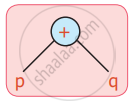Exercise 5.1 | Q 4. (ii) | Page 83

Convert Tree diagram into Algebraic expression.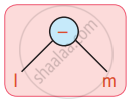Exercise 5.1 | Q 4. (iii) | Page 83

Convert Tree diagram into Algebraic expression.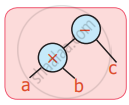Exercise 5.1 | Q 4. (iv) | Page 83

Convert Tree diagram into Algebraic expression.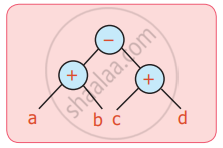Exercise 5.1 | Q 4. (v) | Page 83

Convert Tree diagram into Algebraic expression.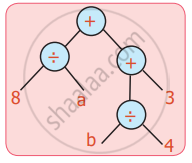Exercise 5.2 [Page 84]

### Tamil Nadu Board Samacheer Kalvi solutions for Class 6th Mathematics Term 2 Answers Guide Chapter 5 Information Processing Exercise 5.2 [Page 84]

#### Miscellaneous practice problems

Exercise 5.2 | Q 1. (i) | Page 84

Write the missing numbers in the tree.

15 × (9 ÷ 3)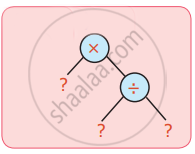Exercise 5.2 | Q 1. (ii) | Page 84

Write the missing numbers in the tree.

65 ÷ (9 + 3)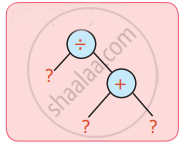Exercise 5.2 | Q 1. (iii) | Page 84

Write the missing numbers in the tree.

(8 + 5) – (9 + 2)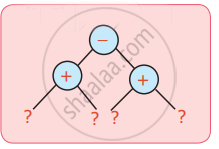Exercise 5.2 | Q 2. (i) | Page 84

Write the missing operations in the tree.

8 + (6 ÷ 2)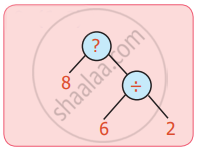Exercise 5.2 | Q 2. (ii) | Page 84

Write the missing operations in the tree.

39 – (6 × 5)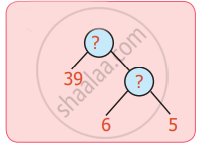Exercise 5.2 | Q 3 | Page 84

Check whether the Tree diagram are equal or not.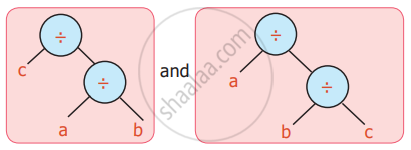#### Challenge problems

Exercise 5.2 | Q 4. (i) | Page 84

Convert the following questions into tree diagram:

The number of people who visited a library in the last 5 months were 1210, 2100, 2550, 3160 and 3310. Draw the tree diagram of the total number of people who had used the library for the 5 months

Exercise 5.2 | Q 4. (ii) | Page 84

Convert the following questions into tree diagram:

Ram had a bank deposit of ₹ 7,55,250 and he had withdrawn ₹ 5,34,500 for educational purpose. Draw a tree diagram for this

Exercise 5.2 | Q 4. (iii) | Page 84

Convert the following questions into tree diagram:

In a cycle factory, 1,600 bicycles were manufactured on a day. Draw tree diagram to find the number of bicycles produced in 20 days

Exercise 5.2 | Q 4. (iv) | Page 84

Convert the following questions into tree diagram:

A company with 30 employees decided to distribute ₹ 90,000 as a special bonus equally among its employees. Draw tree diagram to show how much will each receive?

Exercise 5.2 | Q 5 | Page 84

Write the numerical expression which gives the answer 10 and also convert into tree diagram

Exercise 5.2 | Q 6 | Page 84

Use brackets in appropriate place to the expression 3 × 8 − 5 which gives 19 and convert it into tree diagram for it

Exercise 5.2 | Q 7 | Page 84

A football team gains 3 and 4 points for successive 2 days and loses 5 points on the third day. Find the total points scored by the team and also represent this in tree diagram

## Chapter 5: Information Processing

Exercise 5.1Exercise 5.2## Tamil Nadu Board Samacheer Kalvi solutions for Class 6th Mathematics Term 2 Answers Guide chapter 5 - Information Processing

Tamil Nadu Board Samacheer Kalvi solutions for Class 6th Mathematics Term 2 Answers Guide chapter 5 (Information Processing) include all questions with solution and detail explanation. This will clear students doubts about any question and improve application skills while preparing for board exams. The detailed, step-by-step solutions will help you understand the concepts better and clear your confusions, if any. Shaalaa.com has the Tamil Nadu Board of Secondary Education Class 6th Mathematics Term 2 Answers Guide solutions in a manner that help students grasp basic concepts better and faster.

Further, we at Shaalaa.com provide such solutions so that students can prepare for written exams. Tamil Nadu Board Samacheer Kalvi textbook solutions can be a core help for self-study and acts as a perfect self-help guidance for students.

Concepts covered in Class 6th Mathematics Term 2 Answers Guide chapter 5 Information Processing are Tree Diagram, Conversion of Tree Diagrams into Numerical Expressions, Conversion of Algebraic Expressions into Tree Diagrams.

Using Tamil Nadu Board Samacheer Kalvi Class 6th solutions Information Processing exercise by students are an easy way to prepare for the exams, as they involve solutions arranged chapter-wise also page wise. The questions involved in Tamil Nadu Board Samacheer Kalvi Solutions are important questions that can be asked in the final exam. Maximum students of Tamil Nadu Board of Secondary Education Class 6th prefer Tamil Nadu Board Samacheer Kalvi Textbook Solutions to score more in exam.

Get the free view of chapter 5 Information Processing Class 6th extra questions for Class 6th Mathematics Term 2 Answers Guide and can use Shaalaa.com to keep it handy for your exam preparation